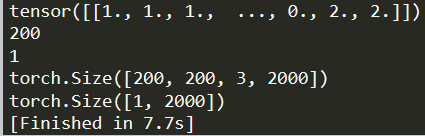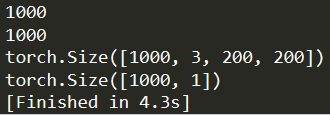# Why is the length of data and label in the read .mat dataset inconsistent?

The length of the data is 200, and the length of the label is 1. These two should correspond to each other! Why is this the case now? Please inform, thank you!

``````import h5py
import torch
from torch.utils.data import TensorDataset

data = tgt_dataset_root['data'][:]
label = tgt_dataset_root['label'][:]

data = torch.from_numpy(data)
label = torch.from_numpy(label)

print(tgt_dataset_root.keys())

print(data)
print(label)

print(len(data))
print(len(label))``````

`len(tensor)` uses the first dimension of the tensor to calculate the length.
Your target seems to have the shape `[1, X]`.
You could use `print(label.size())` to get the size of all dimensions.This is the case, how can I read the length of the next 2000? Or reverse the order of the four columns like the following, these two results show exactly the opposite.It depends how you’ve stored and how you access the HDF5 file.
If the shape is not right, you could `permute` or `view` the tensor to get the right shape.

I tried it. Thank you very much!